JEE  >  JEE Main Physics Mock - 6

# JEE Main Physics Mock - 6

Test Description

## 25 Questions MCQ Test JEE Main & Advanced Mock Test Series | JEE Main Physics Mock - 6

JEE Main Physics Mock - 6 for JEE 2023 is part of JEE Main & Advanced Mock Test Series preparation. The JEE Main Physics Mock - 6 questions and answers have been prepared according to the JEE exam syllabus.The JEE Main Physics Mock - 6 MCQs are made for JEE 2023 Exam. Find important definitions, questions, notes, meanings, examples, exercises, MCQs and online tests for JEE Main Physics Mock - 6 below.
Solutions of JEE Main Physics Mock - 6 questions in English are available as part of our JEE Main & Advanced Mock Test Series for JEE & JEE Main Physics Mock - 6 solutions in Hindi for JEE Main & Advanced Mock Test Series course. Download more important topics, notes, lectures and mock test series for JEE Exam by signing up for free. Attempt JEE Main Physics Mock - 6 | 25 questions in 60 minutes | Mock test for JEE preparation | Free important questions MCQ to study JEE Main & Advanced Mock Test Series for JEE Exam | Download free PDF with solutions
 1 Crore+ students have signed up on EduRev. Have you?
JEE Main Physics Mock - 6 - Question 1

### An ac source is rated at 220V, 50Hz. The time taken for voltage to change from its peak value to zero is

JEE Main Physics Mock - 6 - Question 2

### The ratio of secondary to primary turns is 9:4. If power input (neglect all losses) to power input

JEE Main Physics Mock - 6 - Question 3

### A 5000 kg rocket is set for vertical firing. The exhaust speed is 800 m/s. To give an initial upward acceleration of 20 m/s2, amount of gas ejected per second to supply needed thrust will be (g=10 m/s2)

JEE Main Physics Mock - 6 - Question 4

The energy of a photon of light with wavelength 5000Å is approximately 2.5eV. This way the energy of an X-ray photon with wavelength 1Å would be

JEE Main Physics Mock - 6 - Question 5

Electric field at the centroid of a triangle carrying q charge at each corner is

JEE Main Physics Mock - 6 - Question 6

A particle of mass m is executing uniform circular motion on a path of radius r. If p is the magnitude of its linear momentum. The radial force acting on the particle is

Detailed Solution for JEE Main Physics Mock - 6 - Question 6 Radial force= mv²/r
Multiplying and dividing by m
Force= m²v²/rm
Momentum p= MV
Force = p²/rm
JEE Main Physics Mock - 6 - Question 7
A block of mass M is pulled along a horizontal frictionless surface by a rope of mass m. If a force P is applied at the free end of the rope, then force exerted by the rope on the block is
JEE Main Physics Mock - 6 - Question 8

A particle of mass m and charge q is placed at rest in a uniform electric field E and then released. The kinetic energy attained by the particle after moving a distance y is

JEE Main Physics Mock - 6 - Question 9

Two NOT gates are connected at the two inputs of a NAND gate. This combination will behave like

JEE Main Physics Mock - 6 - Question 10
In a capillary tube, water rises upto 3 mm. The height of water that will rise in another capillary tube having one-third radius of the first is
JEE Main Physics Mock - 6 - Question 11

A body is projected at such an angle that the horizontal range is three times the greatest height. The angle of projection is

JEE Main Physics Mock - 6 - Question 12
If a long hollow copper pipe carries a current, the produced magnetic field will be
JEE Main Physics Mock - 6 - Question 13
In a fission of U235 nucleus on average ----- neutrons are released.
Detailed Solution for JEE Main Physics Mock - 6 - Question 13 When U235 is bombarded by a neutron, it splits into barium,
Krypton and 2 or 3, so average 2.5 neutrons per fission
JEE Main Physics Mock - 6 - Question 14
In the following question, a Statement of Assertion (A) is given followed by a corresponding Reason (R) just below it. Read the Statements carefully and mark the correct answer-
Assertion(A):Physical properties of all isotopes are the same.
Reason(R):Nuclei having same number of protons are all called isotopes.
JEE Main Physics Mock - 6 - Question 15

In the following question, a Statement of Assertion (A) is given followed by a corresponding Reason (R) just below it. Read the Statements carefully and mark the correct answer-
Assertion(A):Two bodies 1 and 2 exerting forces on each other.Force exerted on the body 1 by body 2 is F 12 and that exerted on the body 2 (by body 1) is F 21 . Then F 12 = − F 21 .
Reason(R):Force F 12 is of action and force F 21 is of reaction.

Detailed Solution for JEE Main Physics Mock - 6 - Question 15

Assertion is true as acc to Newton second law Fab=-Fba i.e every action has an equal and opposite reaction. Since force is a Vector quantity opposite direction is to be indicated by negative sign. So in question assertion is true but in reason the force of reaction is not in opposite direction because negative sign is not there. So reason is false.

JEE Main Physics Mock - 6 - Question 16

The M.I. of a body about the given axis is 1.2 kg x m2 initially the body is at rest. In order to produce a rotational kinetic energy of 1500 joule an angular acceleration of 25 rad/sec must be applied about that axis for a duration of

JEE Main Physics Mock - 6 - Question 17
The internal energy of an ideal gas increases during an isothermal process when the gas is
JEE Main Physics Mock - 6 - Question 18

Dimensional formula for torque is

JEE Main Physics Mock - 6 - Question 19
For the wave y = 20 sin π (x/4 + t/2), the correct statement is (where x is in metre and time is in second)
JEE Main Physics Mock - 6 - Question 20

The radionuclide 56Mn is being produced in a cyclotron at a constant rate P by bombarding a manganese target with deutrons. 56Mn has a half life of 2.5 hours and the target contains large number of only the stable manganese isotope 55Mn. The reaction that produces 56Mn is:

55Mn+d→56Mn+P

After being bombarded for a long time, the activity of 56Mn becomes constant equal to 13.86×10s−1. (Use ln2 = 0.693; Avogadro number = 6×1023 atomic weight of 56Mn = 56gm/mole)

Q. After a long time bombardment, number of 56Mn nuclei present in the target depends upon
(a) The number of 56Mn nuclei present at the start of the process
(b) Half life of the 56Mn
(c) The constant rate of production P

*Answer can only contain numeric values
JEE Main Physics Mock - 6 - Question 21

A circular road of radius 1000 m has banking angle 45º. The maximum safe speed of a car having a mass 2000 kg will be, if the coefficient of friction between tyre and road is 0.5 :-

Detailed Solution for JEE Main Physics Mock - 6 - Question 21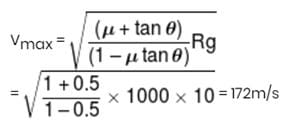*Answer can only contain numeric values
JEE Main Physics Mock - 6 - Question 22

A bob of mass m, suspended by a string of length ℓ1 is given a minimum velocity required to complete a full circle in the vertical plane. At the highest point, it collides elastically with another bob of mass m  suspended by a string of length ℓ2, which is initially at rest. Both the strings are mass-less and inextensible. If the second bob, after collision acquires the minimum speed required to complete a full circle in the vertical plane, the ratio ℓ1/ℓ2 is.

Detailed Solution for JEE Main Physics Mock - 6 - Question 22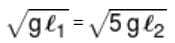*Answer can only contain numeric values
JEE Main Physics Mock - 6 - Question 23

A ring of radius 2m weights 100 kg. Its having pure rolling motion on a horizontal floor so that its centre of mass has a speed of 20 cm/s. If work done to stop it is xJ. Then x will be

Detailed Solution for JEE Main Physics Mock - 6 - Question 23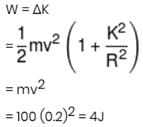*Answer can only contain numeric values
JEE Main Physics Mock - 6 - Question 24

2 kg ice at – 20°C is mixed with 5 kg water at 20°C. Then final amount of water in the mixture would be :
Given specific heat of ice = 0.5cal/g°C, specific heat of water = 1 cal/g°C,  Latent heat of fusion of ice = 80 cal/g. (in kg)

Detailed Solution for JEE Main Physics Mock - 6 - Question 24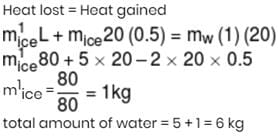*Answer can only contain numeric values
JEE Main Physics Mock - 6 - Question 25

A diode having potential difference 0.5 V across its junction which does not depend on current, is connected in series with resistance of 20 Ω across source. If 0.1 A passes through resistance then what is the voltage of the source :-

Detailed Solution for JEE Main Physics Mock - 6 - Question 25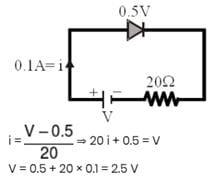## JEE Main & Advanced Mock Test Series

2 videos|325 docs|201 tests
 Use Code STAYHOME200 and get INR 200 additional OFF Use Coupon Code
Information about JEE Main Physics Mock - 6 Page
In this test you can find the Exam questions for JEE Main Physics Mock - 6 solved & explained in the simplest way possible. Besides giving Questions and answers for JEE Main Physics Mock - 6, EduRev gives you an ample number of Online tests for practice

## JEE Main & Advanced Mock Test Series

2 videos|325 docs|201 tests